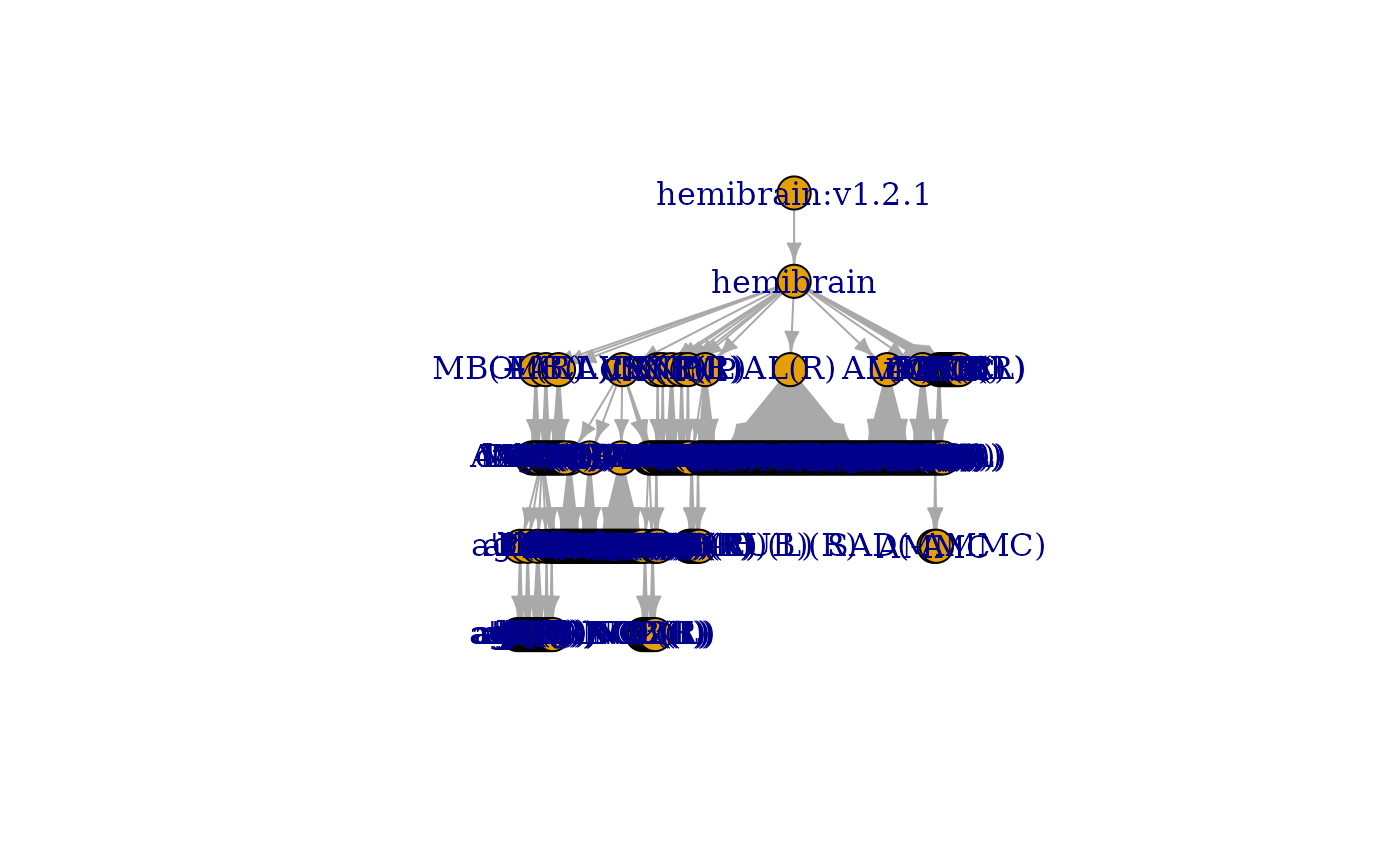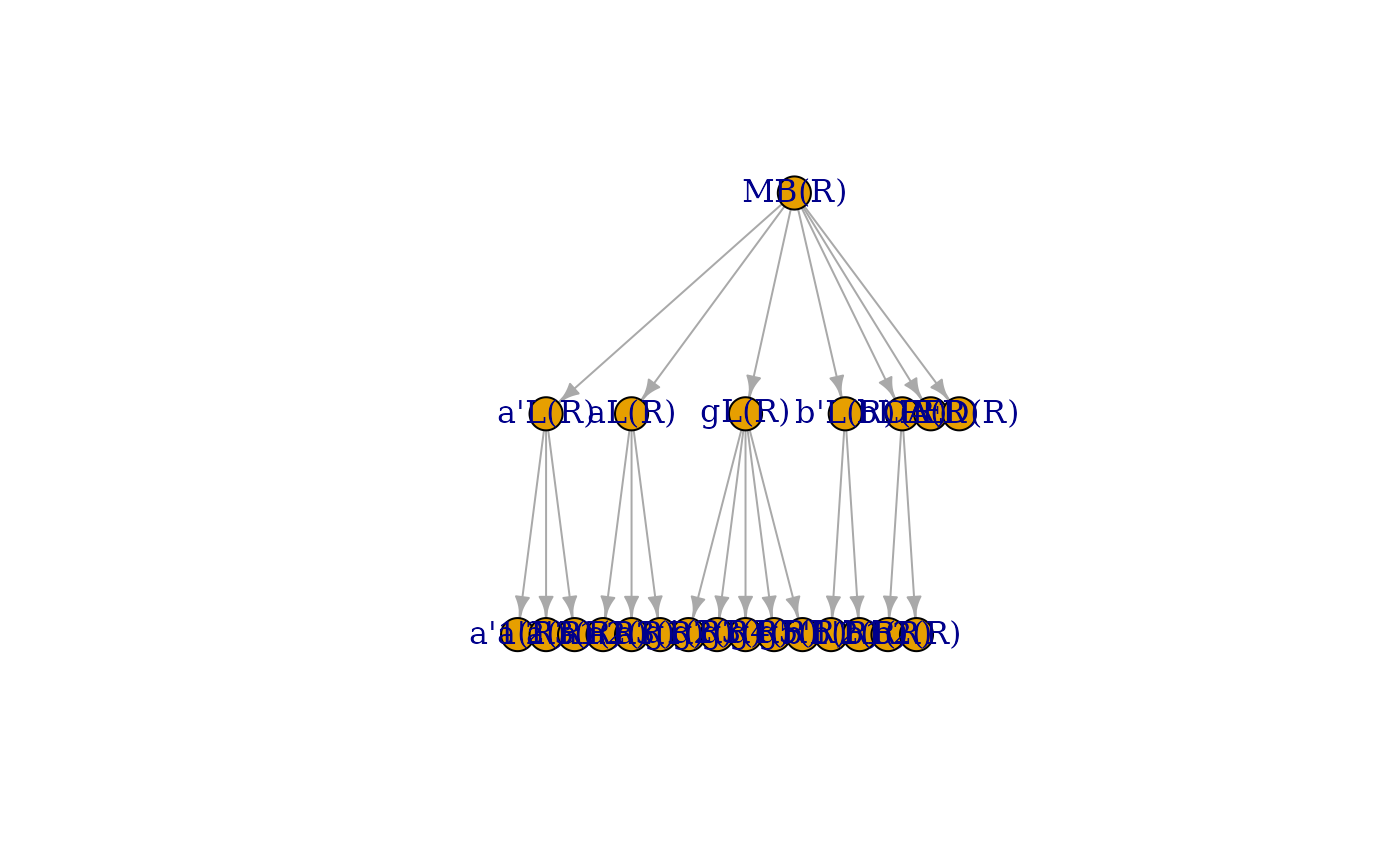Get the region of interest (ROI) hierarchy in a dataset

neuprint_ROI_hierarchy(
root = NULL,
rval = c("edgelist", "graph"),
dataset = NULL,
conn = NULL,
...
)

## Arguments

root

Character vector specifying a root node that defines a subgraph of the ROI hierarchy. The default (root=NULL) will return the whole hierarchy.

rval

Whether to return an edge list data.frame (the default) or an igraph object.

dataset

optional, a dataset you want to query. If NULL, the default specified by your R environ file is used or, failing that the current connection, is used. See neuprint_login for details.

conn

optional, a neuprintr connection object, which also specifies the neuPrint server. If NULL, the defaults set in your .Rprofile or .Renviron are used. See neuprint_login for details.

...

additional arguments passed to neuprint_fetch_custom

neuprint_ROIs, neuprint_get_roiInfo

## Examples

# \donttest{
g = neuprint_ROI_hierarchy(rval='graph')
igraph::plot.igraph(g, layout=igraph::layout_as_tree)# just the Mushroom Body Nodes on right hand side
mbg=neuprint_ROI_hierarchy(root='MB(R)', rval='graph')
igraph::plot.igraph(mbg)# find just the terminal nodes of the graph
mbterms=nat::endpoints(mbg)
mbterms
#>   "CA(R)"  "PED(R)" "a'1(R)" "a'2(R)" "a'3(R)" "a1(R)"  "a2(R)"  "a3(R)"
#>   "g1(R)"  "g2(R)"  "g3(R)"  "g4(R)"  "g5(R)"  "b'1(R)" "b'2(R)" "b1(R)"
#>  "b2(R)"

if (FALSE) {
# read in all the MB meshes
mbmeshes=sapply(mbterms, neuprint_ROI_mesh, simplify = FALSE)
# 3D plot in different colours